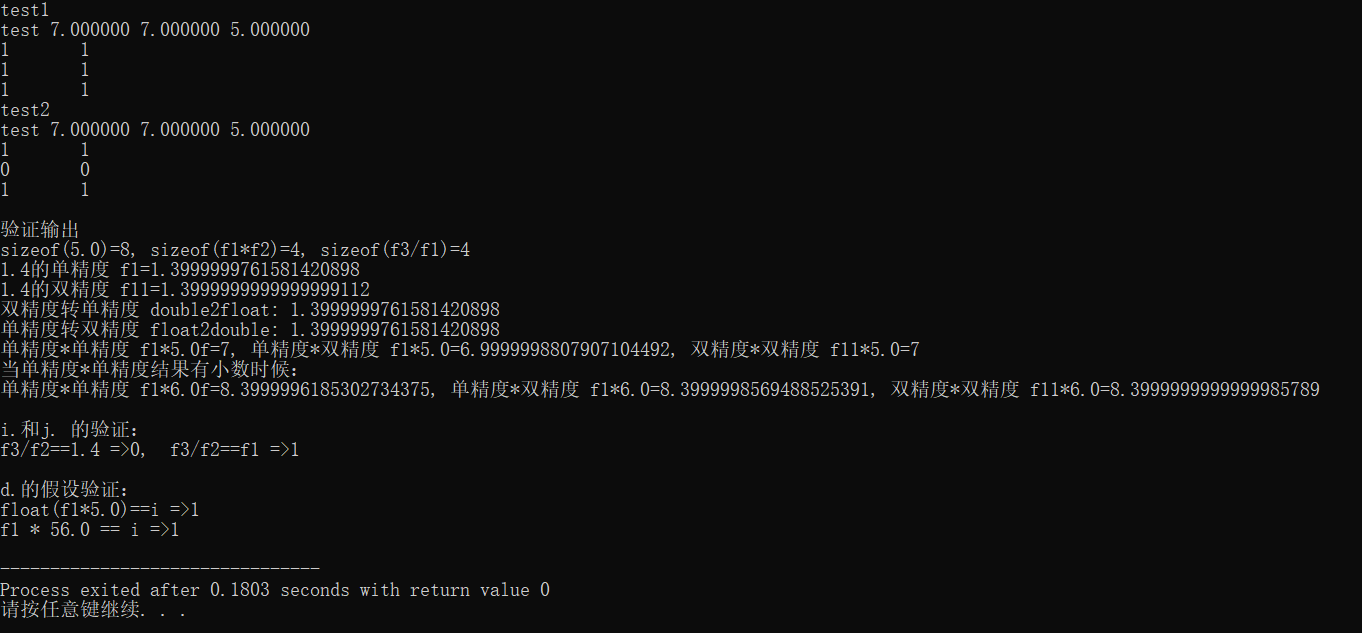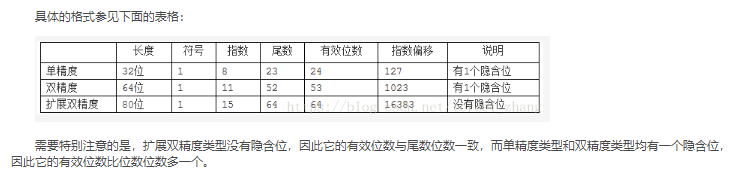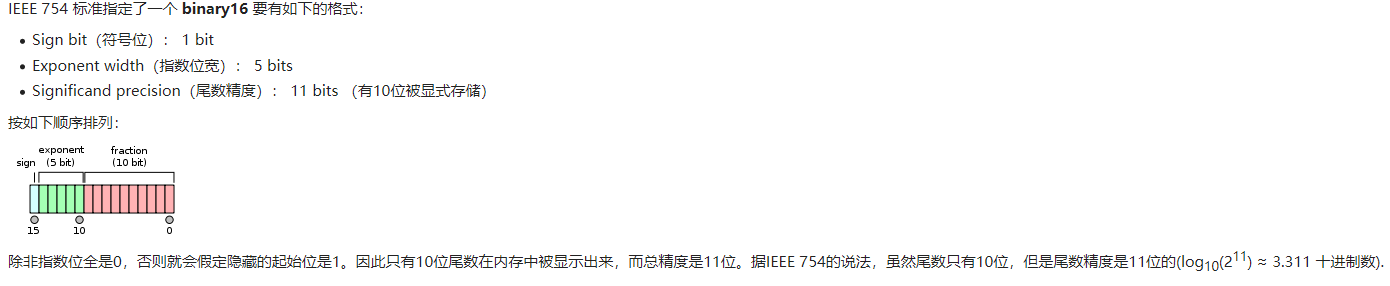• 计算机中float, double类型数据分别占据4，8个字节，其中float类型double可以表示小数位数不同，导致了精度不同。double的精度更高。 计算机中数据表示由：符号位， 指数位，尾数位组成。比如一个float类型...
计算机中float, double类型数据分别占据4，8个字节，其中float类型和double可以表示的小数位数不同，导致了精度不同。double的精度更高。

计算机中数据的表示由：符号位， 指数位，尾数位组成。比如一个float类型数字的二进制由左到右依次是符号位，指数位，尾数位。

类型
符号位
指数位
尾数位
总位数（bit)
float
1
8
23
32
double
1
11
52
64
数字1.4在计算机中的存储转换如下：

先将整数和小数都变二进制表示：1.0110 0110 0110 0110 0110 011，然后整数部分不需要右移，所以float的指数位=2*7-1+0=127；double的指数位=2*10-1+0=1023。各个部分的二进制表示如下。

1.4的不同存储
符号位
指数位
尾数位
16进制表示
float
0
01111111
0110 0110 0110 0110 0110 011
3FB33333
double
0
01111111111
0110 0110 0110 0110 0110 0110 0110 0110 0110 0110 0110 0110 0110
3FF6666666666666

在c++单精度和双精度的运算结合如下两个test示例理解如下：

#include <iostream>

float f1 =1.4;
float f2 = 5.0;
float f3 = 7.0;
int i = 7;
using namespace std;

void test1()
{
printf("test %f %f %f\n", f1 * f2, f1 * 5.0, f3 / f1);
cout << (f1 * f2 == i) << '\t' << (f1 * f2 == f3) << endl;
cout << (f1 * 5.0f == f3) << '\t' << (f1 * f2 == f1 * 5.0f) << endl;
cout << (f3 / f1 == 5.0) << '\t' << (f3 / f1 == f2);
}

void test2()
{
printf("test %f %f %f\n", f1 * f2, f1 * 5.0, f3 / f1);
cout << (f1 * f2 == i) << '\t' << (f1 * f2 == f3) << endl;
cout << (f1 * 5.0 == f3) << '\t' << (f1 * f2 == f1 * 5.0) << endl;
cout << (f3 / f1 == 5.0) << '\t' << (f3 / f1 == f2);
}
int main(void)
{
cout<<"test1"<<endl;
test1();
cout<<endl<<"test2"<<endl;
test2();

cout<<endl<<endl<<"验证输出"<<endl;
double f11=1.4;
cout<<"sizeof(5.0)="<<sizeof(5.0)<<", sizeof(f1*f2)=" <<sizeof(f1*f2)<<", sizeof(f3/f1)=" <<sizeof(f3/f1)<<endl;
cout.precision(20);
cout<<"1.4的单精度 f1="<<f1<<endl<<"1.4的双精度 f11="<<f11<<endl;
cout<<"双精度转单精度 double2float: "<<float(f11)<<endl;
cout<<"单精度转双精度 float2double: "<<double(f1)<<endl;

cout<<"单精度*单精度 f1*5.0f="<<f1*5.0f<<", 单精度*双精度 f1*5.0="<<f1*5.0<<", 双精度*双精度 f11*5.0="<<f11*5.0<<endl;
cout<<"当单精度*单精度结果有小数时候："<<endl;
cout<<"单精度*单精度 f1*6.0f="<<f1*6.0f<<", 单精度*双精度 f1*6.0="<<f1*6.0<<", 双精度*双精度 f11*6.0="<<f11*6.0<<endl;

cout<<endl<<"i.和j. 的验证："<<endl;
cout<<"f3/f2==1.4 =>"<< (f3/f2==1.4)<<",  f3/f2==f1 =>"<<(f3/f2==f1)<<endl;

cout<<endl<<"d.的假设验证："<<endl;
cout<<"float(f1*5.0)==i =>"<<(float(f1*5.0)==i)<<endl;
f1=0.125;
cout<<"f1 * 56.0 == i =>"<< (f1 * 56.0 == i)<<endl;
return 0;

}

test1和test2的两段代码涉及的是float类型和double类型数据在计算机中的存储问题。

用到的基础知识：

1， C++中默认5.0是一个double类型，5.0f表示指定这是一个float类型的数字。

2， 精度（float*double)=> double*double=精度（double), 精度(float*float)=精度(float), 精度(double*double)=精度(double)

3,  float类型用4个字节表示，double类型用8个字节表示，double可以用更多的位表示小数点部分（这部分知识可以参考数字在计算机中的表示，https://blog.csdn.net/ultrakang/article/details/39322275），所以有小数的情况下，double比float精度更高。而float转double精度不变，double转float精度降低。

4，float和double转换中精度降低的情况只作用在存在小数位情况。且只存在于小数位的非0尾数大于23bit情况下（因为float尾数是23个bit, double尾数是52个bit)。

再来看本例中给定的数据 f1=1.4; f2=5.0; f3=7.0; i=7。共进行了如下的运算结果比对，跟着对应的结果分析。

a.  f1 * f2 == i       =》1，左边 f1*f2 等效于 float*float，结果仍然是float，恰好是整数，所以f1 * f2 == i 成立。

b.  f1 * f2 == f3     =》1，左边 同 a. ，右边f3是float类型，不涉及数据类型转换，精度不变，所以f1 * f2 == f3 成立。

c.  f1 * 5.0f == i    =》1，左边 f1*5.0f 等效于float*float，其它同a. ，所以f1 * 5.0f == i  成立 。

d.  f1 * 5.0 == i    =》0，左边 f1*5.0等效于double*double, f1=1.4，1.4作为float和double两种类型在计算机存储的精度是不同的，并且结果也是double类型，精度更高，所以f1 * 5.0 == i 不成立，如果将f1*5.0转换成float类型则成立，即：float(f1*5.0)==i 成立。或者将本例中f1=1.4换成f1=0.125（即一个小数点位数可在23bit之内表示的数 , 则f1 * 56.0 == i 成立，因为0.125值的float和double类型在计算机存储中是一样的。

e.  f1 * f2 == f1 * 5.0f   =》1，右边f1 * 5.0f是float*float，不涉及类型转换问题，同b. 所以f1 * f2 == f1 * 5.0f 成立。

f.   f1 * f2 == f1 * 5.0   =》0，右边同d. 1.4在计算机中作为float和double表示的精度不同，f1*5.0是double*double=double，比f1*f2的float*float=float精度更高，所以f1 * f2 == f1 * 5.0不成立。

g.  f3 / f1 == 5.0     =》1,  左边float/foat=float，结果用float类型表示，小数位尾数为0，右边double类型的5.0的小数位尾数也都是0，所以f3 / f1 == 5.0 成立。可以结合下面的 i. 来理解。

h.  f3 / f1 == f2      =》1， 左边和右边都是float类型，精度不变，所以 f3 / f1 == f2成立。可以结合下面的j. 来理解。

补充：

i.  f3 / f2 == 1.4   =》0, 左边结果float类型，右边1.4是double类型，精度不同，所以f3 / f2 == 1.4不成立。

j. f3 / f2 == f1   =》1，右边类型是float，1.4用float表示，不涉及精度降低问题，所以f3 / f2 == f1 成立。

cout默认打印出7位有效数字，所以第一行打印的结果都一样。

上面的验证结果如图：展开全文c++ 数据结构 float double
• 126) * 0.1 = 2**(-127) 0 00000000 00000000000000000000001 = +1 * 2**(-126) * 0.00000000000000000000001 = 2**(-149) (Smallest positive value) 双精度 IEEE双精度浮点标准表示需要64位字，其可以从左到右表示...
注意：Nintendo 64有一个64位处理器，但是：Many games took advantage of the chip’s 32-bit processing mode as the greater data precision available with 64-bit data types is not typically required by 3D games, as well as the fact that processing 64-bit data uses twice as much RAM, cache, and bandwidth, thereby reducing the overall system performance.The term double precision is something of a misnomer because the precision is not really double.The word double derives from the fact that a double-precision number uses twice as many bits as a regular floating-point number.For example, if a single-precision number requires 32 bits, its double-precision counterpart will be 64 bits long.The extra bits increase not only the precision but also the range of magnitudes that can be represented.The exact amount by which the precision and range of magnitudes are increased depends on what format the program is using to represent floating-point values.Most computers use a standard format known as the IEEE floating-point format.单精度IEEE单精度浮点标准表示需要一个32位字，从左到右可以表示为从0到31编号。>第一位是符号位，S，>接下来的8位是指数位，’E’和>最后23位是分数’F’：S EEEEEEEE FFFFFFFFFFFFFFFFFFFFFFF0 1      8 9                    31由字表示的值V可以如下确定：>如果E = 255且F为非零，则V = NaN(“不是数字”)>如果E = 255且F为零，S为1，则V = -Infinity>如果E = 255且F为零，S为0，则V =无穷大>如果0意在表示通过用F加前缀而创建的二进制数隐式前导1和二进制点。>如果E = 0且F为非零，则V =( – 1)** S * 2 **(-126)*(0.F)。这些是“非规范化”值。>如果E = 0且F为零，S为1，则V = -0>如果E = 0并且F为零并且S为0，则V = 0尤其是，0 00000000 00000000000000000000000 = 01 00000000 00000000000000000000000 = -00 11111111 00000000000000000000000 = Infinity1 11111111 00000000000000000000000 = -Infinity0 11111111 00000100000000000000000 = NaN1 11111111 00100010001001010101010 = NaN0 10000000 00000000000000000000000 = +1 * 2**(128-127) * 1.0 = 20 10000001 10100000000000000000000 = +1 * 2**(129-127) * 1.101 = 6.51 10000001 10100000000000000000000 = -1 * 2**(129-127) * 1.101 = -6.50 00000001 00000000000000000000000 = +1 * 2**(1-127) * 1.0 = 2**(-126)0 00000000 10000000000000000000000 = +1 * 2**(-126) * 0.1 = 2**(-127)0 00000000 00000000000000000000001 = +1 * 2**(-126) *0.00000000000000000000001 =2**(-149)  (Smallest positive value)双精度IEEE双精度浮点标准表示需要64位字，其可以从左到右表示为从0到63编号。>第一位是符号位，S，>接下来的11位是指数位，’E’和>最后52位是分数’F’：S EEEEEEEEEEE FFFFFFFFFFFFFFFFFFFFFFFFFFFFFFFFFFFFFFFFFFFFFFFFFFFF0 1        11 12                                                63由字表示的值V可以如下确定：>如果E = 2047且F为非零，则V = NaN(“不是数字”)>如果E = 2047且F为零，S为1，则V = -Infinity>如果E = 2047，F为零，S为0，则V =无穷大>如果0意在表示通过用F加前缀而创建的二进制数隐式前导1和二进制点。>如果E = 0且F为非零，则V =( – 1)** S * 2 **(-1022)*(0.F)是“非规范化”值。>如果E = 0且F为零，S为1，则V = -0>如果E = 0并且F为零并且S为0，则V = 0参考：ANSI / IEEE标准754-1985，二进制浮点算术标准。
展开全文• 3.浮点运算加法乘法 3.1加法 3.2乘法 1.实数数表示 参考深入理解C语言-03-有符号数，定点数，浮点数 1.1定点数 一般在没有FPU寄存器嵌入式系统中使用比较多。比如常见32位系统中，将高16位作为整数...
目录

1.实数数的表示

1.1定点数

1.2浮点数

2.精度说明

2.1半精度FP16

3.浮点运算加法和乘法

3.1加法

3.2乘法

1.实数数的表示

参考深入理解C语言-03-有符号数，定点数，浮点数

1.1定点数

一般在没有FPU寄存器的嵌入式系统中使用比较多。比如常见的32位系统中，将高16位作为整数部分，低16位作为小数部分。
这样就可以用整数来模拟定点数的 +  - * / 运算。
关于定点数的数学分析，请参考以下文档：http://www.digitalsignallabs.com/fp.pdf

代码实现可以参考以下文档：http://www.eetimes.com/author.asp?section_id=36&doc_id=1287491

1.2浮点数

格式：

s符号位  exp指数   frac尾数   精度说明

16位       1            5             11            半精度 FP16
32位       1            8             23            单精度 FP32
64位       1           11            52            双精度 FP64
11位       1            4             6              没找到应用 11bit存储起来也奇怪

表示的数为： (-1)的s次方 *  2的(exp -base)次方 * (1 + frac)
base = 2的(exp位数 -1) -1 对于32位，为127 = 2的7次方 -1

比如0.325 =1.3 / 4  （规范化，这种方式在信息处理中很常见）
则s为0， exp为 127 + (-2) = 125, frac为0.3
近一步把0.3表示为  1/4 + 1/20 = (1/4) * ( 1 + 1/5)
注意到1/5可以表示为 (3/16) / (1 - 1/16) = (3/16) ( 1 + 1/16 + (1/16)*(1/16) + ... (无穷级数展开)
对应的二进制表示为
0.  01  (0011) (0011) ...
取前23位，则为：
0100110 01100110 01100110

这样，0.325在内存中的2进制表示为：
0  01111101  0100110 01100110 01100110

对应16进制为：3E A6 66 66

2.精度说明

半精度 16bit，单精度32bit，双精度64，上文已经提出，需要注意的是FP16，FP32，FP64都有隐藏的起始位。参考程序员必知之浮点数运算原理详解

以半精度FP16为例说明

2.1半精度FP163.浮点运算加法和乘法

相比于整数加法和乘法多了比较，移位逻辑，比整数复杂很多

3.1加法

浮点加法器首先对浮点数拆分，得到符号、阶码、尾数。对拆分结果进行绝对值比较，得到大的阶码、阶差和比较结果输出。然后进行对阶，通过移位小的尾数，得到相同大阶。对尾数进行尾数加减运算，得到的结果进行规格化，最后结合规格化结果运算结果符号输出，得到结果输出。

一.需要注意一个是以绝对值大的对阶数(exponent), 二.对结果规格化规划到(1+Frac)的形式。

3.2乘法

符号位只是两者的异或，指数位基本上是两指数的相加，而尾数位就需要在正常的乘法之余考虑到移位（和随之而来的指数为的溢出）和进位的情况。


展开全文• 实际上，任何精度的浮点运算也可以像BigInteger那样计算，可以用多个浮点数据表示更高精度的浮点数据。而这就要求实现带余数的浮点四则运算。 带余数的浮点四则运算的定义： 加法减法，余数结果的能精确表示...
实际上，任何精度的浮点运算也可以像BigInteger那样计算，可以用多个浮点数据表示更高精度的浮点数据。而这就要求实现带余数的浮点四则运算。

带余数的浮点四则运算的定义：

加法和减法，余数和结果的和能精确表示运算值。

乘法和除法，余数和结果必须同号，对于乘法，余数和结果的和能精确表示运算值，而对于除法，余数就是原来的意思。

不多解释，代码如下：（修复某些可能存在错误）

#include <iostream>
#include <xmmintrin.h>

using namespace std;

typedef struct {
float hi;
float lo;
} doublf;

/**
* BigFloat的实际值为：
* 使用这个数据类型的缺点是数据存储冗余，优点是便于计算。
**/
typedef struct {
int32_t len;//data中有效数据的长度
int32_t exp;//指数部分
float data;//浮点数据，每个都是1.x的数据
} BigFloat;

inline int32_t ifabs(float a){
int32_t p=*(int32_t*)&a;
return p&0x7fffffff;
}

//o=5
doublf addf(float a,float b){//带余数加法
doublf ret;
ret.hi=a+b;
if(ifabs(a)>ifabs(b))ret.lo=b-(ret.hi-a);
else ret.lo=a-(ret.hi-b);
return ret;
}

//o=5
doublf subf(float a,float b){//带余数减法
doublf ret;
ret.hi=a-b;
if(ifabs(a)>ifabs(b))ret.lo=(a-ret.hi)-b;
else ret.lo=a-(ret.hi+b);
return ret;
}

//o=14
doublf mule(float a,float b){
doublf ret;
float m,n;//结合整数运算优化
int32_t ap,bp,am,bm;
uint32_t mp;
ap=*(int32_t*)&a;
bp=*(int32_t*)&b;
ret.hi=n=a*b;
am=ap&0xff800000;
bm=bp&0xff800000;
am=am+bm-0x4b000000;
m=*(float*)&am;
ap|=0x800000;
bp|=0x800000;
mp=(uint32_t)ap*(uint32_t)bp;
ap=(mp&0x7fffff)|am;
ret.lo=*(float*)&ap;
if(mp&0x800000){
if(n+m!=n)ret.lo-=m;
}
else ret.lo-=m;
return ret;
}

//o=14
doublf mulf(float a,float b){//带余数乘法
unsigned int csr=_mm_getcsr();
_mm_setcsr((csr & ~_MM_ROUND_MASK) | _MM_ROUND_TOWARD_ZERO);
doublf ret=mule(a,b);
_mm_setcsr(csr);
return ret;
}

//o=17
doublf divf(float a,float b){//带余数除法
unsigned int csr=_mm_getcsr();
_mm_setcsr((csr & ~_MM_ROUND_MASK) | _MM_ROUND_TOWARD_ZERO);
doublf ret,tmp;
ret.hi=a/b;
tmp=mule(ret.hi,b);
ret.lo=(a-tmp.hi)-tmp.lo;
_mm_setcsr(csr);
return ret;
}

//检验正确
//o=20,8
doublf add(doublf a,doublf b){//最後一位会产生误差
doublf ret,tmp;
/* 计算 */
if(ifabs(a.hi)<ifabs(b.hi)){
tmp=a;
a=b;
b=tmp;
}
ret=addf(b.lo,a.lo);
tmp=addf(ret.hi,b.hi);
ret.hi=tmp.hi;
ret.lo+=tmp.lo;
tmp=addf(tmp.hi,a.hi);
ret.hi=tmp.hi;
ret.lo+=tmp.lo;//即使高低位重叠也不会有影响
return ret;
}

//检验正确
//o=22,9
doublf sub(doublf a,doublf b){//最後一位会产生误差
doublf tmp;
/* 计算 */
tmp.hi=-b.hi;
tmp.lo=-b.lo;
return add(a,tmp);
}

//检验正确
doublf mul(doublf a,doublf b){
#if 0//最後一位会产生误差
//o=137,25
unsigned int csr=_mm_getcsr();
_mm_setcsr((csr & ~_MM_ROUND_MASK) | _MM_ROUND_TOWARD_ZERO);
doublf ret,tmp,tmp2,tmp3,tmp4;
/* 计算 */
tmp=mule(a.lo,b.lo);
tmp2=mule(a.lo,b.hi);
tmp3=mule(a.hi,b.lo);
tmp4=mule(a.hi,b.hi);
_mm_setcsr(csr);
ret=add(tmp,tmp2);//应允许误差存在
ret=add(ret,tmp3);
ret=add(ret,tmp4);
return ret;
#else//最後两位会产生误差
//o=25,8
doublf ret=mulf(a.hi,b.hi);
ret.lo+=(a.lo*b.lo+a.lo*b.hi)+a.hi*b.lo;
ret=addf(ret.hi,ret.lo);//克服高低位重叠造成的影响
return ret;
#endif
}

//检验正确
doublf div(doublf a,doublf b){//最後两位会产生误差
#if 1//o=27,10
doublf ret,tmp;
float d=b.hi+b.lo;
ret.hi=a.hi/d;
unsigned int csr=_mm_getcsr();
_mm_setcsr((csr & ~_MM_ROUND_MASK) | _MM_ROUND_TOWARD_ZERO);
/* 计算 */
tmp=mule(b.hi,ret.hi);
//tmp2=mule(b.lo,ret.hi);
_mm_setcsr(csr);
ret.lo=((((a.hi-tmp.hi)-tmp.lo)-ret.hi*b.lo)+a.lo)/d;//精度低但更快
//a=sub(a,tmp);
//a=sub(a,tmp2);
//ret.lo=a.hi/d;
ret=addf(ret.hi,ret.lo);//还是应该修正，以克服高低位重叠的影响
return ret;
#else//o=24,8
doublf ret;
float d=b.hi+b.lo;
ret=divf(a.hi,d);
ret.lo=(ret.lo-ret.hi*((d-b.hi)-b.lo)+a.lo)/d;
return ret;
#endif
}

inline double to_double(doublf a){
return (double)a.hi+a.lo;
}

inline doublf to_doublf(double a){
doublf ret;
ret.hi=a;
ret.lo=a-ret.hi;
return ret;
}

/**
* 这样就能有办法用单精度模拟双精度（可以有2^-46的精度），甚至模拟任何精度的浮点数据。
* 关键是要支持带余数的浮点四则运算。
**/

int main()
{
int i;
uint64_t tmp;
doublf df,af,tf;
double dd=45671.154545,ad=1.001245648454,td,tt;
do{
tf=df=to_doublf(dd);
af=to_doublf(ad);
cin>>i;
while(i--){
tt=to_double(df=add(df,af));
tmp=*(uint64_t*)&tt;
cout<<"add="<<hex<<tmp<<endl;
td=dd+ad;
tmp=*(uint64_t*)&td;
cout<<"chk="<<hex<<tmp<<endl;
dd=tt;
};
df=tf;
dd=to_double(tf);
cin>>i;
while(i--){
tt=to_double(df=sub(df,af));
tmp=*(uint64_t*)&tt;
cout<<"sub="<<hex<<tmp<<endl;
td=dd-ad;
tmp=*(uint64_t*)&td;
cout<<"chk="<<hex<<tmp<<endl;
dd=tt;
};
df=tf;
dd=to_double(tf);
cin>>i;
while(i--){
tt=to_double(df=mul(df,af));
tmp=*(uint64_t*)&tt;
cout<<"mul="<<hex<<tmp<<endl;
td=dd*ad;
tmp=*(uint64_t*)&td;
cout<<"chk="<<hex<<tmp<<endl;
dd=tt;
};
df=tf;
dd=to_double(tf);
cin>>i;
while(i--){
tt=to_double(df=div(df,af));
tmp=*(uint64_t*)&tt;
cout<<"div="<<hex<<tmp<<endl;
td=dd/ad;
tmp=*(uint64_t*)&td;
cout<<"chk="<<hex<<tmp<<endl;
dd=tt;
};
cin>>dd;
cin>>ad;
}while(ad!=0.0);
return 0;
}



展开全文算法 c++
• 计算机处理数据都涉及到数据转换各种复杂运算，比如，不同单位换算，不同进制(如二进制十进制)换算等，很多除法运算不能除尽，比如10÷3=3.3333.。。。。。。无穷无尽，而精度是有限，3.3333333x3并不等于10，...
• 关于16进制字符串转为单精度浮点数和双精度浮点数的运算(一)最近在进行GPIB方面的开发时，传回的数据有这两种格式，1.IEEE 32位浮点数格式；2.IEEE 64位浮点数格式。1.IEEE 32位浮点数格式 这种格式的特点是：每个...16进制 float 16进制转float
• 计算机处理数据都涉及到数据转换各种复杂运算，比如，不同单位换算，不同进制(如二进制十进制)换算等，很多除法运算不能除尽，比如10÷3=3.3333.。。。。。。无穷无尽，而精度是有限，3.3333333x3并不等于10，...
• 大致原因是M40是单精度的浮点数的运算，P4是双精度，在双精度上的计算结果用在单精度上运行，运算精度不一样，所以计算偏差比较大，导致的结果不一致。特别是乘除法的矩阵运算，结果运算偏差会很大。 解决方式是模型...
• 这种格式特点是：每个数由8字节组成，包括1位符号位，11位带符号阶码，52位尾数。 例如：我们收到一个数格式是 3F F0 6F 80 00 00 00 00，那么它二进制格式是： 0011 1111 1111 0000 0110 1111 1000 0000 ...16进制 转double
•  作为美国国家半导体LMP高精度放大器系列中新成员，最新推出LMP7731组装与LMP7732组装高精度运算放大器输入电压噪声只有2.9nV/sqrtHz，中频噪声转角仅为3Hz，而0Hz～10Hz峰值噪声电压也只有78nVpp，...
• ## STM32双精度运算

千次阅读 2013-11-28 22:03:48
调试程序的时候，用到两个双精度的运算，我写的公式比较长，这部分的计算竟然影响了中断部分的执行。具体点说，就是由于双精度运算，影响了进入跳出中断定时器的执行。如果我直接用假数，进入中断都是正常的；换成...
• 　作为美国国家半导体LMP高精度放大器系列中新成员，最新推出LMP7731组装与LMP7732组装高精度运算放大器输入电压噪声只有2.9nV/sqrtHz，中频噪声转角仅为3Hz，而0Hz～10Hz峰值噪声电压也只有78nVpp，...
•  作为美国国家半导体LMP高精度放大器系列中新成员，最新推出LMP7731组装与LMP7732组装高精度运算放大器输入电压噪声只有2.9nV/sqrtHz，中频噪声转角仅为3Hz，而0Hz～10Hz峰值噪声电压也只有78nVpp，...
• 当前NVIDAGPU芯片仅支持单精度(float)浮点运算，对一些应用来说精度可能不够用，一些关键步骤可能需要双精度运算，才能保证程序正常执行。对此，本人尝试用两个单精度浮点数数来代表一个双精度浮点数：//类型...
• float double 互相运算的时候，建议将float转换为double后统一类型运算。 float转换double时会造成精度丢失，我一个解决办法是，将float转换为string，再转换为double 如： floatf=3.141...
• 此外，补码与原码相互转换，其运算过程是相同，不需要额外硬件电路。 正整数补码是其二进制表示，与原码相同。 https://blog.csdn.net/jiaobuchong/article/details/83188674补码原理个人理解...
• 在C/C++中float是32位，double是64位，两者在内存中存储方式能够表示的精度均不同，目前C/C++编译器标准都遵照IEEE制定浮点数表示法来进行float,double运算。 无论是float还是double，在内存中存储...C
• 美国国家半导体公司(National Semiconductor Corporation)宣布推出5款高精度运算放大器，即使增益操作超过6V/V，也可领信号调节和传感器接口保持极高准确度，非常适合对高精度和高增益要求较高应用，如便携式...
• 前面把原始的C代码转成了ISPC可编译的C代码，其中image_rotate_double_ispc函数里面的数据都是基于double双精度来运算的。我的电脑是支持AVX/AVX2指令集的，所以一次可以并发做4个double浮点的运算，理论上可以提升4...图像处理
• float double 互相运算的时候，建议将float转换为double后统一类型运算。float转换double时会造成精度丢失，我一个解决办法是，将float转换为string，再转换为double如：floatf=3.141592Fdoubled=Convert.To...
• 目前C/C++ 编译器标准都遵照IEEE 制定浮点数表示法来进行float,double 运算。这种结构是一种科学计数法，用符号、指数尾数来表示，底数定为2—— 即把一个浮点数表示为尾数乘以2 指数次方再添上符号。...
• 基础知识： 十进制转十六进制；...这种结构是一种科学计数法，用符号、指数尾数来表示，底数定为2—— 即把一个浮点数表示为尾数乘以2 指数次方再添上符号。下面是具体规格：  符号位CC++
• 　作为美国国家半导体LMP高精度放大器系列中新成员，最新推出LMP7731组装与LMP7732组装高精度运算放大器输入电压噪声只有2.9nV/sqrtHz，中频噪声转角仅为3Hz，而0Hz-10Hz峰值噪声电压也只有78nVpp，因此...
• 单精度双精度浮点存储表示 基础知识： 十进制转十六进制； 十六进制转二进制； 了解： 目前C/C++编译器标准都遵照IEEE制定浮点数表示法来进行float,double运算。这种结构是一种科学计数法，用符号、指数...
• 通道CS3004CS3014、通道CS30033013集成电路具有比其他同类运算放大器更加优异的精度。此外，CS3013CS3014所具备低功耗特性，对燃气安全检测器等新一代电池供电便携仪器个人监测应用具有重要意义...
• 　通道CS3004CS3014、通道CS30033013集成电路具有比其他同类运算放大器更加优异的精度。此外，CS3013CS3014所具备低功耗特性，对燃气安全检测器等新一代电池供电便携仪器个人监测应用具有重要意义...
• 　通道CS3004CS3014、通道CS30033013集成电路具有比其他同类运算放大器更加优异的精度。此外，CS3013CS3014所具备低功耗特性，对燃气安全检测器等新一代电池供电便携仪器个人监测应用具有重要意义......